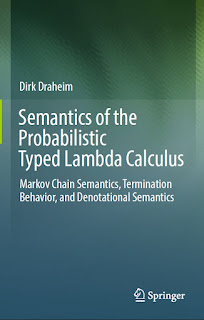CALCULUS MATHEMATICS

# Semantics of the Probabilistic Typed Lambda Calculus Markov Chain Semantics, Termination Behavior, and Denotational Semantics Free DownloadThis book takes a foundational approach to the semantics of probabilistic programming. It elaborates a rigorous Markov chain semantics for the probabilistic typed lambda calculus, which is the typed lambda calculus with recursion plus probabilistic choice. The book starts with a recapitulation of the basic mathematical tools needed throughout the book, in particular Markov chains, graph theory and domain theory, and also explores the topic of inductive definitions. It then defines the syntax and establishes the Markov chain semantics of the probabilistic lambda calculus and, furthermore, both a graph and a tree semantics. Based on that, it investigates the termination behavior of probabilistic programs. It introduces the notions of termination degree, bounded termination and path stop ability and investigates their mutual relationships. Lastly, it defines a denotational semantics of the probabilistic lambda calculus, based on continuous functions over probability distributions as domains.